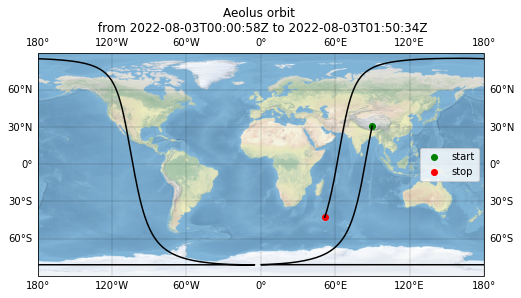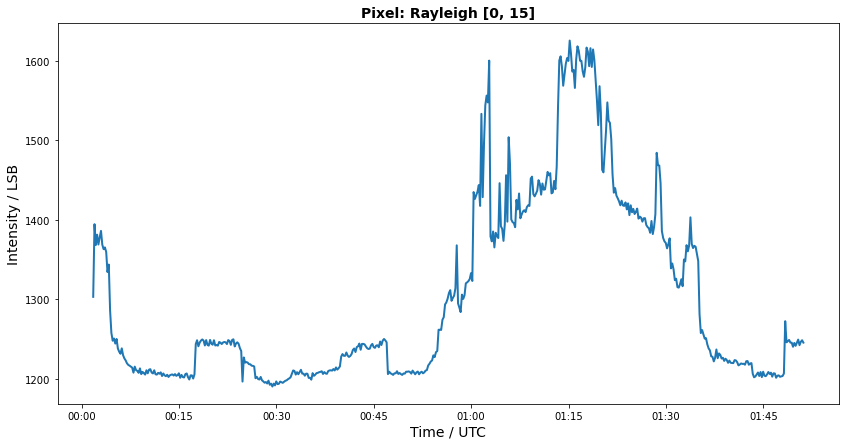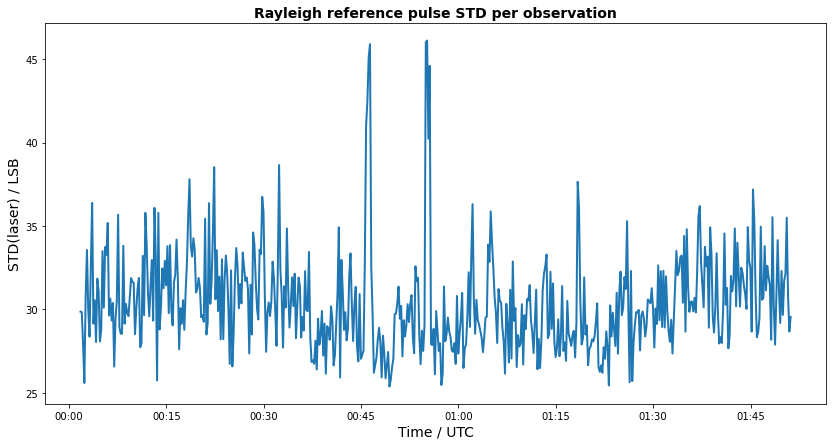# Aeolus Level 1A product

## Contents

from viresclient import AeolusRequest
import matplotlib.pyplot as plt
import matplotlib.dates as mdates
from netCDF4 import Dataset
import pandas as pd
from netCDF4 import num2date


# Aeolus Level 1A product¶

## Product information¶

The Level 1A product of the Aeolus mission contains time-ordered unprocessed detector counts from the atmospheric and internal instrument paths as well as housekeeping information.

### L1A parameters on VirES¶

We can print the available parameters for a collection using the print_available_collections method.

request = AeolusRequest()
request.print_available_collections(collection="ALD_U_N_1A")

uom description
field type identifier
observation_fields time seconds since 2000-01-01 UTC Time at center of obs/meas.
mie_longitude degrees_east Longitude of each Mie height bin along the line-of-sight
mie_latitude degrees_north Latitude of each Mie height bin along the line-of-sight
mie_altitude m Altitude of Mie height bins with respect to the WGS84 ellipsoid along the line-of-sight
mie_topocentric_azimuth_of_height_bin degrees Azimuth of target-to-satellite topocentric pointing vector of the height bin
mie_topocentric_elevation_of_height_bin degrees Elevation of target-to-satellite topocentric pointing vector of the height bin
mie_target_to_sun_visibility_flag -
mie_range m Distance along the LOS between the instrument and the lower edge of the Mie height bin
rayleigh_longitude degrees_east Longitude of each Rayleigh height bin along the line-of-sight
rayleigh_latitude degrees_north Latitude of each Rayleigh height bin along the line-of-sight
rayleigh_altitude m Altitude of Rayleigh height bins with respect to the WGS84 ellipsoid along the line-of-sight
rayleigh_topocentric_azimuth_of_height_bin degrees Azimuth of target-to-satellite topocentric (horizontal reference frame) pointing vector of the height bin
rayleigh_topocentric_elevation_of_height_bin degrees Elevation of target-to-satellite topocentric (horizontal reference frame) pointing vector of the height bin
rayleigh_target_to_sun_visibility_flag - Sun visibility flag. Value set to -1 if sun eclipsed by earth; value set to +1 if sun (center) visible
rayleigh_range m Distance along the LOS between the instrument and the lower edge of the Rayleigh height bin
latitude_of_DEM_intersection degrees_north Latitude of the intersection of DEM and the line-of-sight
longitude_of_DEM_intersection degrees_east Longitude of the intersection of DEM and the line-of-sight
altitude_of_DEM_intersection m Altitude of the intersection of DEM and the line-of-sight
argument_of_latitude_of_dem_intersection degrees_north Argument of latitude of DEM intersection
sun_elevation_angle deg Sun elevation angle at DEM intersection
velocity_at_DEM_intersection m/s Velocity along the line of sight at the DEM
geoid_separation m Height of geoid above WGS84 ellipsoid
AOCS_x_position m X position in Earth-fixed reference
AOCS_y_position m Y position in Earth-fixed reference
AOCS_z_position m Z position in Earth-fixed reference
AOCS_x_velocity m/s X velocity in Earth-fixed reference
AOCS_y_velocity m/s Y velocity in Earth-fixed reference
AOCS_z_velocity m/s Z velocity in Earth-fixed reference
AOCS_roll_angle degrees Roll angle in satellite reference for each observation
AOCS_pitch_angle degrees Pitch angle in satellite reference for each observation
AOCS_yaw_angle degrees Yaw angle in satellite reference for each observation
num_reference_pulses - Number of reference pulses
mie_reference_pulse - Contains pixel values. The first 2 values are the pre-pixel of the ACCD. The last two are used to correct the offset.
rayleigh_reference_pulse - Contains pixel values. The first 2 values are the pre-pixel of the ACCD. The last two are used to correct the offset
instrument_mode - Instrument mode. Laser burst warm up, calibration slow, calibration fast 1, calibration fast 2
number_of_pulses_per_meas - Number of pulses per measurement
number_of_meas_per_obs - Number of measurements per BRC
average_laser_frequency_offset GHz Average laser frequency offset
average_laser_energy mJ Average laser pulse UV energy of N * (P - 1) pulses, regardless of the UV_Energy_OK_Status
laser_frequency_offset_std_dev GHz Laser frequency offset standard deviation
uv_energy_std_dev mJ Laser pulse UV energy standard deviation
dt1 - dt1 pulse delay constant for BRC
dt2 - dt2 pulse delay constant for BRC
dt3_fixed - Fixed dt3 pulse delay constant for BRC
dt4 - dt4 pulse delay constant for BRC
dt5 - dt5 pulse delay constant for BRC
deu_imaging_integration_time - DEU Imaging Integration Time for the IDC mode
td_ray_mie - Td_ray_mie
mie_bin_integration_time - Individual time delay for each layer (bin)
mie_bkg_integration_time - Duration of background integration time
ray_bin_integration_time - Individual time delay for each layer (bin)
ray_bkg_integration_time - Duration of background integration time
mie_accd_temp degC Average of 10 Mie ACCD die temperatures
ray_accd_temp degC Average of 10 Rayleigh ACCD die temoeratures
deu_temp degC Average of DEU temperature
m1_temp degC Average of Aht 22, Aht 23, Aht 24, Aht 25, Aht 26 and Aht 27 M1 temperatures
aht_22_tel_m1 degC Average of Aht 22 M1 temperatures
aht_23_tel_m1 degC Average of Aht 23 M1 temperatures
aht_24_tel_m1 degC Average of Aht 24 M1 temperatures
aht_25_tel_m1 degC Average of Aht 25 M1 temperatures
aht_26_tel_m4 degC Average of Aht 26 M1 temperatures
aht_27_tel_m5 degC Average of Aht 27 M1 temperatures
m1_tc_temp degC Average of TC 18, TC 19, TC 20, and TC 21 M1 TC temperatures
tc_18_tel_m11 degC Average of TC 18 Tel M1-1 temperatures
tc_19_tel_m12 degC Average of TC 19 Tel M1-2 temperatures
tc_20_tel_m13 degC Average of TC 20 Tel M1-3 temperatures
tc_21_tel_m14 degC Average of TC 21 Tel M1-4 temperatures
tc_25_tm15_ths1y degC Average of TC 25 TM15 Ths1-Y temperatures
tc_27_tm16_ths1y degC Average of TC 27 TM16 Ths1-Y temperatures
tc_29_ths2 degC Average of TC 29 Ths2 temperatures
tc_23_ths1 degC Average of TC 23 Ths1 temperatures
tc_32_ths3 degC Average of TC 32 Ths3 temperatures
struts_temp_pxpy degC Average of Struts temperature +X+Y
struts_temp_mxpy degC Average of Struts temperature -X+Y
struts_temp_mpy degC Average of Struts temperature -Y
m2_tc_temp degC Average of M2 TC temperature
mie_measurement_data - Mie measurement data, restructured to be as obs, meas, layer, pixel
rayleigh_measurement_data - Rayleigh measurement data, restructured to be as obs, meas, layer, pixel
measurement_fields time seconds since 2000-01-01 UTC Time at center of obs/meas.
AOCS_x_position m X position in Earth-fixed reference
AOCS_y_position m Y position in Earth-fixed reference
AOCS_z_position m Z position in Earth-fixed reference
AOCS_x_velocity m/s X velocity in Earth-fixed reference
AOCS_y_velocity m/s Y velocity in Earth-fixed reference
AOCS_z_velocity m/s Z velocity in Earth-fixed reference
AOCS_roll_angle degrees Roll angle in satellite reference for each measurement
AOCS_pitch_angle degrees Pitch angle in satellite reference for each measurement
AOCS_yaw_angle degrees Yaw angle in satellite reference for each measurement
mie_longitude degrees_east Longitude of each Mie height bin along the line-of-sight
mie_latitude degrees_north Latitude of each Mie height bin along the line-of-sight
mie_altitude m Altitude of Mie height bins with respect to the WGS84 ellipsoid along the line-of-sight
mie_range m Distance along the LOS between the instrument and the lower edge of the Mie height bin
rayleigh_longitude degrees_east Longitude of each Rayleigh height bin along the line-of-sight
rayleigh_latitude degrees_north Latitude of each Rayleigh height bin along the line-of-sight
rayleigh_altitude m Altitude of Rayleigh height bins with respect to the WGS84 ellipsoid along the line-of-sight
rayleigh_range m Distance along the LOS between the instrument and the lower edge of the Rayleigh height bin
latitude_of_DEM_intersection degrees_north Latitude of the intersection of DEM and the line-of-sight
longitude_of_DEM_intersection degrees_east Longitude of the intersection of DEM and the line-of-sight
altitude_of_DEM_intersection m Altitude of the intersection of DEM and the line-of-sight
argument_of_latitude_of_dem_intersection degrees_north Argument of latitude of DEM intersection
sun_elevation_angle deg Sun elevation angle at DEM intersection
velocity_at_DEM_intersection m/s Velocity along the line of sight at the DEM

## Defining product, parameters and time for the data request¶

Keep in mind that the time for one full orbit is around 90 minutes. The repeat cycle of the orbits is 7 days.

# Aeolus product
DATA_PRODUCT = "ALD_U_N_1A"

# measurement period in yyyy-mm-ddTHH:MM:SS
measurement_start="2022-08-03T00:00:58Z"
measurement_stop="2022-08-03T01:50:34Z"

# Product parameters to retrieve
# uncomment parameters of interest

# Mie observation level
parameter_mie_observations = [
"reference_pulse",
"measurement_data",
"longitude",
"latitude",
]

parameter_mie_observations = ["mie_" + param for param in parameter_mie_observations]

# Rayleigh observation level
parameter_rayleigh_observations = [
"reference_pulse",
"measurement_data",
"longitude",
"latitude"
]

parameter_rayleigh_observations = ["rayleigh_" + param for param in parameter_rayleigh_observations]

parameter_observations_indepdendent = ["time", "num_reference_pulses"]

parameter_list_observations = (
parameter_rayleigh_observations
+ parameter_mie_observations
+ parameter_observations_indepdendent
)


## Retrieve data from VRE server¶

# Data request for observation level
# check if observation parameter list is not empty
if len(parameter_list_observations) > 0:

request = AeolusRequest()

request.set_collection(DATA_PRODUCT)

# set observation fields
request.set_fields(
observation_fields=parameter_list_observations,
)

# set start and end time and request data
data_response = request.get_between(
start_time=measurement_start, end_time=measurement_stop, filetype="nc", asynchronous=False

)

ds_observations = data_response.as_xarray()


## Plot data on map¶

import cartopy.crs as ccrs

fig = plt.figure(figsize=(8, 6))
ax = fig.add_subplot(1, 1, 1, projection=ccrs.PlateCarree())
ax.stock_img()
gl = ax.gridlines(draw_labels=True, linewidth=0.3, color="black", alpha=0.5, linestyle="-")
ax.plot(
ds_observations["rayleigh_longitude"][:, -1],
ds_observations["rayleigh_latitude"][:, -1],
"k-",
transform=ccrs.PlateCarree(),
)
ax.scatter(
ds_observations["rayleigh_longitude"][0, -1],
ds_observations["rayleigh_latitude"][0, -1],
marker="o",
c="g",
edgecolor="g",
label="start",
transform=ccrs.PlateCarree(),
)
ax.scatter(
ds_observations["rayleigh_longitude"][-1, -1],
ds_observations["rayleigh_latitude"][-1, -1],
marker="o",
c="r",
edgecolor="r",
label="stop",
transform=ccrs.PlateCarree(),
)
ax.legend()
ax.set_title("Aeolus orbit \n from {} to {}".format(measurement_start, measurement_stop))

Text(0.5, 1.0, 'Aeolus orbit \n from 2022-08-03T00:00:58Z to 2022-08-03T01:50:34Z')## Plot ACCD counts at pixel level¶

The following example allows to visualize the raw ACCD counts of the atmospheric path for selected pixels. The data is averaged over the measurements of the observations.

# select channel and pixel coordinate (counting starts at zero)

channel = 'rayleigh'
pixel = [0, 15]

if not channel in ('mie', 'rayleigh'):
raise Exception('Invalid channel selection. Allowed values are "mie" or "rayleigh"')

if not 0 <= pixel <= 24:
raise Exception('Invalid pixel selection. Allowed range for the pixel row is from 0 to 24.')

if not 0 <= pixel <= 15:
raise Exception('Invalid pixel selection. Allowed range for the pixel column is from 0 to 15.')

# extract data
pixel_data = ds_observations[channel + '_measurement_data'][:, :, pixel, pixel].data.mean(axis=1)
time = num2date(
ds_observations["time"], units="s since 2000-01-01", only_use_cftime_datetimes=False
)

fig = plt.figure(figsize=(14, 7))
ax = plt.subplot()
ax.plot(time, pixel_data, linewidth=2.0)
ax.set_title('Pixel: ' + channel.capitalize() + ' ' + str(pixel), fontsize=14, fontweight='bold')
ax.set_xlabel('Time / UTC', fontsize=14)
ax.set_ylabel('Intensity / LSB', fontsize=14)
ax.xaxis.set_major_formatter(mdates.DateFormatter("%H:%M"))## Visualize reference pulse data¶

The following example demonstrates how to analyse reference pulse data by visualizing the standard deviation of the sunmmed up reference signal counts per observation.

# select channel
channel = 'rayleigh'

if not channel in ('mie', 'rayleigh'):
raise Exception('Invalid channel selection. Allowed values are "mie" or "rayleigh"')

# extract data
num_ref_pulse = ds_observations["num_reference_pulses"][:].data
ref_pulse_std = ds_observations[channel + '_reference_pulse'][:, :num_ref_pulse, 2:18].data.sum(axis=2).std(axis=1)

fig = plt.figure(figsize=(14, 7))
ax = plt.subplot()
ax.plot(time, ref_pulse_std, linewidth=2.0)
ax.set_title(channel.capitalize() + ' reference pulse STD per observation', fontsize=14, fontweight='bold')
ax.set_xlabel('Time / UTC', fontsize=14)
ax.set_ylabel('STD(laser) / LSB', fontsize=14)
ax.xaxis.set_major_formatter(mdates.DateFormatter("%H:%M"))